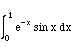Trapezoid rule using MATLAB

Determine the value of the following integral.In MATLAB create a M-file f.m.

function y=f(x)
y = exp(-x).*sin(x);

The .* is used because this function will be evaluated for a vector, x, so that exp(­x) and sin(x) form vectors. Then what is wanted is the elements of exp(­x) and sin(x) multiplying each other, to give the element in the vector y. This is what the .* does.

Then create a vector of x values:

»x=0:.1:1

 0 0.1 0.2 0.3 0.4 0.5 0.6 0.7 0.8 0.9 1

Then create a vector of y values.

»y = f(x)

 0 0.09033 0.16266 0.21893 0.26103 0.29079 0.30988 0.31991 0.32233 0.31848 0.30956

Check that the y values are correct by calculating one of them. Finally calculate the area.

»area = trapz(x,y)

area = 0.24491148225216

This is the answer if only 11 points are used. Increase the number to 101, 201, and 401.

»x=0:.01:1;

»y=f(x);

»area = trapz(x,y)

area = 0.24582775039918

»x = 0:.005:1;

»y=f(x);

»area = trapz(x,y)

area = 0.24583469284741

»x = 0:.0025:1;

»y=f(x);

»area = trapz(x,y)

area = 0.24583642846187

These values can be used in a Romberg exptrapolation, as shown in the Table.

Table. Values of Integral using Trapezoid Rule
 n area 101 0.24582775039918 201 0.24583469284741 401 0.24583642846187

Taking the two answers obtained with the smallest x gives

I = 4*(0.24583642846187 ­ 0.24583469284741) / 3 = 0.24583700700002

If all values are used we get:

 0.24582775039918 0.24583469284741 0.245836 0.24583700699682 0.245837 0.245837

These extrapolated values are all accurate enough, since they achieve 9 digit accuracy even though the original values had at most 5 digit accuracy.